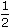# Aptitude - Area - Discussion

### Discussion :: Area - General Questions (Q.No.6)

6.

The diagonal of the floor of a rectangular closet is 7feet. The shorter side of the closet is 4feet. What is the area of the closet in square feet?

[A].
 5 1 4
[B].
 13 1 2
[C]. 27
[D]. 37

Explanation:

Other side =152 -92 2 2
ft
=
 225 - 81 4 4
ft
=
 144 4
ft
= 6 ft.Area of closet = (6 x 4.5) sq. ft = 27 sq. ft.

 Jagan said: (Jan 28, 2011) What is closet mean?

 Bhargav said: (Jun 13, 2011) How 4.5 came ?

 Kiran Joy said: (Jun 17, 2011) Closet only means a closed space. So here in a rectangle diagonal=7. 5 feet and breadth=4. 5 feet. So using hypotenuse2=base2+altitude2 we can easily find the lenght. So area= l*b.

 Divya said: (Jul 11, 2011) Shorter side( width) = 4 1/2 = 9/2= 4.5 other side(length) = 6 Area= 6*4.5 = 27

 Siddhesh said: (Aug 10, 2013) Please give the answer for how 4.5 came?

 Vaishnavi said: (Sep 12, 2013) @Siddhesh. In the question itself one of the side value is given that is 4*1^2 (shortest closet). Since it s shortest closet we are taking that as breadth value 4.5 feet and derived value as the length (6 feet).

 Surinder Singh said: (Dec 21, 2013) What is the shape of closet?

 Gaurav said: (Mar 21, 2014) (Hypotenuse)2 = (Base)2 + (Height)2. So! H^2 = hy^2 - Base^2 ----- eq1. Here hy = 15/2 , base= 9/2 ...................(*9/2 = 4.5). After solve eq1, we get 6ft Area of closet = 6ft * 4.5 ft (this come from base, see to * ..).

 Sheela said: (Jun 5, 2014) Using the formula given hy^2=base^2+hgt^2. We get the ans as 6ft and here in the question we have to find the area in square feet so we have to multiply with 4.5 = 9/2 so we have the ans of 27 with the product of 4.5 and 6 because we are considering the side but not the diagonal.

 Sheela said: (Jun 5, 2014) Using the formula given hy^2=base^2+hgt^2. We get the ans as 6ft and here in the question we have to find the area in square feet so we have to multiply with 4.5 = 9/2 so we have the ans of 27 with the product of 4.5 and 6 because we are considering the side but not the diagonal.

 Kuldeep Kumar said: (Mar 13, 2015) Length of closet will come 12/2 feet. (Using Pythagoras theory). And area = l*b = (9/2)*(12/2) = 9*3 = 36 sq.feet. Then how it comes 27 sq.feet?

 Shri said: (Apr 13, 2015) @Siddhesh. Breadth i.e. the smallest side is 9/2 ie 4.5 since the area of rectangle is length*breadth therefore the final step is 6*9/2 or 6*4.5

 Zafar said: (May 23, 2015) If suitable sketches were given and hypotenuse theorem is to be applied on required statement, learners got it easy.

 Rabab said: (May 30, 2015) How 6 is coming? Can you simplify?

 Sravani said: (Jul 13, 2015) Once look at the explanation at the top, I hope you will get.

 Dheerendra said: (Feb 9, 2016) Shouldn't closet mean a cuboid or a cube?

 Mathslover said: (Mar 14, 2016) A closet is a little room where clothes are hung. This closet is in the shape of a rectangle. The diagonal of the floor of the closet is 7.5 ft and the shorter side is 4.5 ft. To find the area of the floor we need the measure of the longer side. Remember that when we have a diagonal in a rectangle or square we will have 2 triangles. Now using the Pythagoras Theorem: a^2 + b^2 = c^2. 4.5^2 +b^2 =7.5^2. b^2 = 7.5^2 - 4.5^2. b^2 = 56.25^2 - 20.25^2. b^2 = 36. Therefore b = 6 (length of longer side). Area = length x width. = 6 x 4.5. = 27ft^2.

 Sivaram said: (Mar 8, 2018) You correct @Sheela. By using Pythagoras theory, we can predict.

 Gourikrishna said: (Sep 21, 2019) How 15/2 and 9/2 came? Explain me in clear.

 Captain said: (Apr 23, 2020) Your explanation is best, Thanks @Kiran.

 Punni said: (May 30, 2020) @Kiran Joy. Thank You so much. Now the logic of the question is clear.